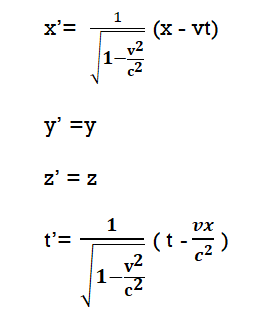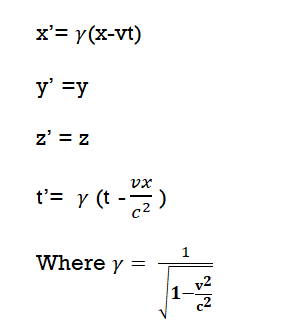Goseeko blog# What is Lorentz Transformation?

## Introduction

Lorentz transformation gives the relationship between two different coordinate frames moving at a constant velocity w.r.t. to each other. This name of the transformation is given on the name of the scientist Dutch physicist Hendrik Lorentz who discovered it.

## Need of  Lorentz Transformation

The Galilean transformation nevertheless violates Einstein’s postulates. Because it is found that light moving with speed c along the x-axis would travel at speed c−v in the other inertial frame. This violates the velocity equation state.

The laws of mechanics are invariant under Galilean transformations. Whereas Maxwell’s equations and electrodynamics laws are varying under this transformation. This shows that for different observers moving with different uniform velocities. The velocity of light will have different values. But the speed of light is invariant in all inertial frames of reference. Hence, Galilean transformations needs modification.

Thus We need a transformation between two inertial frames which

• Preserves the velocity of light.
• Keeps the forms of laws of physics invariant.

Hence, Lorentz transformation equations achieved this. Lorentz deduced the transformation equations. These equations are in agreement with the results of Michelson–Morley experiment.

The Lorentz transformations can written in following way. Where x, y, z, t are position and time coordinate in frame S and x’, y’, z’, t’  are position and time coordinate in frame S’ frame which is moving with velocity v w.r.t. frame S.

These equations therefore areThis given set of equations, relating the position and time in the two inertial frames is Lorentz transformation

The reverse transformation is expressed by the following equations. Simply interchanging the primed and unprimed variables and substituting gives:This given set of equations, relating the position and time in the two inertial frames is the Reverse Lorentz transformation

Interested in learning about similar topics? Here are a few hand-picked blogs for you!